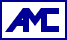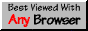# Kong: The network delay ape

• Source code: kong-source.tar.gz (14 kB, released 2003-Jul-19-Sat)
See also the source code for Tangle, specifically the files rttdata*, delayer*, rng*, and complainer* in the src/ directory.
• Measurements: kong-data-2003-04.tar (98 MB, collected 2003-Apr)
(The archive is not compressed because the individual members are.)

In the course of working on the Tangle project, the need arose to simulate end-to-end network delays. Since communication in Tangle is connectionless, intermittent, and very low-bandwidth, there was no need to simulate the network topology; all I needed was the end-to-end delays. I looked around for a model, but could not find one that people had confidence in.

I did, however, find a tool for measuring network delays: `king`, by Krishna P. Gummadi et al. It measures the round-trip time (RTT) between DNS servers. Since most DNS servers are willing to perform recursive queries, it is possible to measure the RTT between two remote points; there is no need to install instrumentation on machines scattered around the internet.

This suggested a solution to my problem: Choose N points at random on the internet, measure the RTT between every pair, and mimic those RTTs (“ape” them) in the simulation.

Actually, I wanted more than a single number for each pair; I wanted a probability distribution. That could be achieved by collecting many sample RTTs for each pair, at different times. These can be sorted, filtered, and interpolated into a smooth commulative distribution function, which can be used to generate random delays. Since the communication is intermittent, we can neglect time dependence and generate each delay independently.

But there was a scaling problem: Measuring every pair among N nodes would require O(N^2) time, and storing the data would require O(N^2) space. But a simulation of N nodes typically requires only O(N (log N)^k) time and space (that is true for Tangle), so this simple solution would quickly become the limiting factor of the simulation.

This problem was overcome by introducing one level of recursion. Let n = sqrt(N), and measure every pair among n points. Now the data takes O(n^2) = O(N) time to collect and O(n^2) = O(N) space to store. In the simulation, imagine that each of the n nodes is a gateway to a local region, which looks like a scaled-down copy of the whole n-node cloud. There are now (n)(n-1) = N (almost) simulated endpoints, and the total RTT between two endpoints is the sum of three segments (two local and one wide-area) unless the two endpoints happen to be in the same local region. The scale factor for region i is the ratio of the min and max of the median RTTs between node i and all the other nodes, which is just enough to ensure that between any pair of endpoints neither local segment median is greater than the wide-area segment median.

Of course this model is bogus; the internet does not really look like that. But since the scale factors tend to be large, the local segments tend to be small compared to the wide-area segments, so whatever interesting phenomena were measured in the n-node cloud (like violations of the triangle inequality) will presumably still be true in the simulated N-node cloud. In any case, something had to be done to address the scalability problem, otherwise the whole approach is useless.

The available `king` tool is designed to measure one RTT. I needed to measure more than a million, so DNS server failures were inevitable, and needed to be retried. But transient failures needed to be distinguished from persistent failures, because retries are slow and waste a lot of time. I ended up writing a new program, `kingpairs`, from scratch, using the same core technique as king. I also wrote various scripts to drive `kingpairs` and process the output. All of that code, and the data collected in 2003-Apr, are available via the links at the top of this page.

For the code that generates the simulated delays based on the data, see the Tangle project.Prepared by Adam M. Costello  Last modified: 2004-Jan-06-Tue 20:13:38 GMT